# 12-240/Homework Assignment 4

(diff) ← Older revision | Latest revision (diff) | Newer revision → (diff)

This assignment is due at the tutorials on Thursday October 18. Here and everywhere, neatness counts!! You may be brilliant and you may mean just the right things, but if the teaching assistants will be having hard time deciphering your work they will give up and assume it is wrong.

Task 2. Solve problems 3, 8, 9, 10, and 11 on pages 41-42, but submit only your solutions of problems 8, 9, and 11.

Task 3. Solve problems 1, 2, 4, 5, 9, 12, 13, and 16 on page 53-56, but submit only your solutions of problems 4, 5, 9, and 12.

 Just for Fun (1). Take a large integer and write it in base 10. Cut away the "singles" digit, double it and subtract the result from the remaining digits. Repeat the process until the number you have left is small. Prove that the number you started from is divisible by 7 iff the resulting number is divisible by 7. Thus the example on the right shows that 86415 is divisible by 7 as 0 is divisible by 7. Find a similar criterion for divisibility by 17 and for all other divisibilities and indivisibilities. Note that the word "indivisibilities" has the largest number of repetitions of a single letter among all words in the English language (7 i's). I've known this fact for many years yet here's a semi-legitimate use for that word! (It is tied with the word honorificabilitudinitatibus for seven 'i's. You can read more about it here: http://en.wikipedia.org/wiki/Honorificabilitudinitatibus) ```86415 10 ---- 8631 2 --- 861 2 -- 84 8 - 0 ```

Just for Fun (2). Is there a problem with the following inductive proof that all horses are of the same color?

We assert that in all sets with precisely$n$ horses, all horses are of the same color. For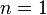$n=1$, this is obvious: it is clear that in a set with just one horse, all horses are of the same color. Now assume our assertion is true for all sets with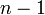$n-1$ horses, and let us be given a set with$n$ horses in it. By the inductive assumption, the first$n-1$ of those are of the same color and also the last$n-1$ of those. Hence they are all of the same color as illustrated below: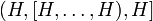$(H,[H,\ldots,H),H]$

(The horses surrounded by round brackets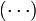$(\cdots)$ are all of the same color. The horses surrounded by square brackets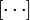$[\cdots]$ are all of the same color. Therefore the first and the last horses have the same color as the ones in the middle group, and hence all horses are of the same color.)